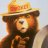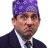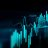# Convert ThreeXOscillator ThinkScript to MetaTrader 4?

M

#### Mamba10

##### New member
Hey guys,

This maybe the opposite of what many people may ask on this forum and I am not sure if anyone here can help, but I was wondering if anyone can assist me in converting the ThreeXOscillator from ThinkScript to MetaTrader4? Is it possible?
Here is the code:

Code:
``````# RH_Three_X_OscillatorSTUDY.ts

# Code by rhouser

# Permission granted to use, copy, modify to members of the Yahoo TOS_thinkscript Group for personal use only

#hint:<b>RH_Three_X_Oscillator</b>\nThis is the companion study to the 9/30 Setup. It plots a Stochastic at three times the current timeframe, and the Stochastic at the current timeframe

declare lower;

input threeX_K_period = 21;

input threeX_D_period = 9;

input threeXsmoothing = 3;

input oneX_K_period   = 14;

input oneX_D_period   = 3;

input oneXsmoothing   = 3;

input smoothing_type  = AverageType.SIMPLE;

input threeXstochType = { FAST, default SLOW };

input oneXstochType   = { FAST, default SLOW };

input over_bought     = 75;

input over_sold       = 25;

input maxLookback     = 3;

def BarNum            = if IsNaN( close ) then Double.NaN else BarNumber();

def aggPer            = getAggregationPeriod();

def adjAggPer        = if aggPer == AggregationPeriod.MIN then

AggregationPeriod.THREE_MIN

else if aggPer == AggregationPeriod.TWO_MIN then

AggregationPeriod.FIVE_MIN

else if aggPer == AggregationPeriod.THREE_MIN then

AggregationPeriod.TEN_MIN

else if aggPer == AggregationPeriod.FOUR_MIN then

AggregationPeriod.TEN_MIN

else if aggPer == AggregationPeriod.FIVE_MIN then

AggregationPeriod.FIFTEEN_MIN

else if aggPer == AggregationPeriod.TEN_MIN then

AggregationPeriod.THIRTY_MIN

else if aggPer == AggregationPeriod.FIFTEEN_MIN then

AggregationPeriod.HOUR

else if aggPer == AggregationPeriod.TWENTY_MIN then

AggregationPeriod.HOUR

else if aggPer == AggregationPeriod.THIRTY_MIN then

AggregationPeriod.TWO_HOURS

else if aggPer == AggregationPeriod.HOUR then

AggregationPeriod.FOUR_HOURS

else if aggPer == AggregationPeriod.TWO_HOURS then

AggregationPeriod.DAY

else if aggPer == AggregationPeriod.FOUR_HOURS then

AggregationPeriod.DAY

else if aggPer == AggregationPeriod.DAY then

AggregationPeriod.THREE_DAYS

else if aggPer == AggregationPeriod.TWO_DAYS then

AggregationPeriod.WEEK

else if aggPer == AggregationPeriod.THREE_DAYS then

AggregationPeriod.WEEK

else if aggPer == AggregationPeriod.FOUR_DAYS then

AggregationPeriod.MONTH

else if aggPer == AggregationPeriod.WEEK then

AggregationPeriod.MONTH

else if aggPer == AggregationPeriod.MONTH then

AggregationPeriod.MONTH

else

Double.NaN;

def _kPeriod            = threeX_K_period;

def _dPeriod            = threeX_D_period;

def _threeXsmoothing    = threeXsmoothing;

def threeXhighestHigh = highest( high( period = adjAggPer ), _kPeriod );

def threeXlowestLow   = lowest( low( period = adjAggPer ), _kPeriod );

def threeXfastK       = if ( threeXhighestHigh - threeXlowestLow ) <= 0 then 0 else 100 * ( close( period = adjAggPer ) - threeXlowestLow ) / ( threeXhighestHigh - threeXlowestLow );

def threeXfastD       = MovingAverage( smoothing_type, threeXfastK, _dPeriod );

def threeXslowK       = threeXfastD;

def threeXslowD       = MovingAverage( smoothing_type, threeXslowK, _dPeriod );

def oneXfastK         = if ( highest( high, oneX_K_period ) - lowest( low, oneX_K_period ) ) <= 0

then 0

else 100 * ( close - lowest( low, oneX_K_period ) ) / ( highest( high, oneX_K_period ) - lowest( low, oneX_K_period ) );

def oneXfastD         = MovingAverage( smoothing_type, oneXfastK, oneX_D_period );

def oneXslowK         = oneXfastD ;

def oneXslowD         = MovingAverage( smoothing_type, oneXslowK , oneX_D_Period );

#---Stochastic

plot ThreeX_Momentum  = if threeXstochType == threeXstochType.FAST then threeXfastD else threeXslowD;

plot OneX_Trend       = if oneXstochType   == oneXstochType.FAST   then oneXfastD   else oneXslowD;

#---Reference lines

plot OverBought       = over_bought;

plot OverSold         = over_sold;

def state             = { default init, up, down };

switch( state ) {

case init:

if barNum == 1 then {

state           = state.init;

} else {

state           = if ThreeX_Momentum > ThreeX_Momentum

then state.up

else if ThreeX_Momentum < ThreeX_Momentum

then state.down

else state.init;

}

case up:

state             = if ThreeX_Momentum >= ThreeX_Momentum

then state.up

else state.down;

case down:

state             = if ThreeX_Momentum <= ThreeX_Momentum

then state.down

else state.up;

}

#===============================[ Look & Feel ]================================

ThreeX_Momentum.SetPaintingStrategy( PaintingStrategy.LINE_VS_POINTS );

ThreeX_Momentum.AssignValueColor( if state == state.up then Color.Dark_ORANGE else if state == state.down then Color.BLUE else Color.GRAY );

ThreeX_Momentum.HideBubble();

OneX_Trend.SetPaintingStrategy( PaintingStrategy.LINE );

OneX_Trend.AssignValueColor( if OneX_Trend >= OneX_Trend then Color.MAGENTA else if OneX_Trend < OneX_Trend then Color.DARK_RED else Color.GRAY );

OneX_Trend.HideBubble();

OverBought.SetDefaultColor( Color.LIGHT_GRAY );

OverBought.HideTitle();

OverSold.SetDefaultColor( Color.DARK_GRAY );

OverSold.HideTitle();

def Hundred           = 100;

AddCloud( OverBought, Hundred, Color.RED, Color.RED );

def Zero              = 0;

AddCloud( OverSold, Zero, Color.Yellow, Color.Yellow );``````

Last edited by a moderator:
J

#### JoeSD

##### New member
VIP
Mamba, I tried loading your ThreeXOscillator code into TOS and did NOT work. Do you have a study link that you could share with me that actually shows on TOS? It looks like an interesting indicator and I'd like to try it out. If anyone else can help that would be great too. Thanks.

•dougnHow do I convert this ATR% chart study into a scanner watchlist column? Questions 4Convert encoded Mt4 to Thinkscript Questions 2Convert Chande Kroll Stop to ThinkorSwim Questions 2